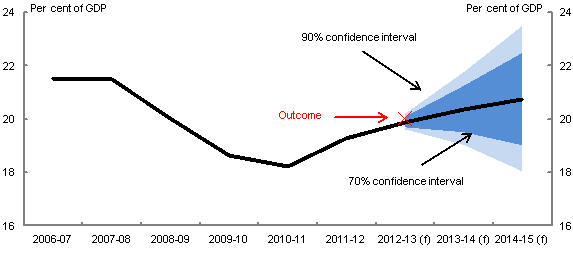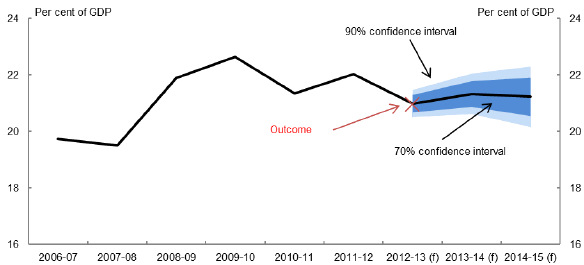# Appendix B: Alternative to no-GDP-error approach

Date

ï»¿

There is more than one way to calculate confidence intervals which reflect uncertainty in the level of fiscal variable but not in nominal GDP. Apart from the no-GDP-error approach used above, an alternative is to base the confidence intervals on the root mean squared errors of the cumulative growth rate forecasts of the level of the fiscal variables. Using this approach, the root mean squared errors are used to first derive confidence intervals around the cumulative growth rates of the fiscal variables (similar to what was done in Charts 1 and 2) and then these confidence intervals can be used to calculate confidence intervals for the levels of the fiscal variables and hence confidence intervals for the fiscal variables as a share of GDP (by dividing the confidence intervals for the levels by the forecast for nominal GDP). This cumulative growth rate approach can be used for receipts and payments but it is problematic to use for the underlying cash balance, as the growth rate and forecast errors will be large if the underlying cash balance is close to zero.13

The results using this cumulative growth rate approach (Charts B1 and B2) are similar to those based on the no-GDP-error approach (Charts 7 and 8). They suggest that, at the time of the 2013-14 Budget, the widths of the 70 per cent confidence intervals for forecasts for 2014-15 were roughly \$60 billion for receipts and \$20 billion for payments.

Chart B1: Receipts (excluding GST) as a percentage of GDP

(Cumulative growth rate approach)Note: The central line shows the outcomes and point estimate forecasts in the 2013-14 Budget. Confidence intervals use root mean squared errors (RMSE) for Budget forecasts from the 1998-99 Budget onwards. RMSE do not reflect forecast errors caused by policy decisions taken after the relevant Budget. These confidence intervals are derived from the cumulative growth rate forecast errors of the level of the fiscal variable and so do not reflect uncertainty about nominal GDP. (f) are forecasts. Before the 2009-10 Budget, a projection rather than a forecast was made for the year after the budget year. The outcome is based on information available at the time of the Final Budget Outcome.

Source: Budget papers and Treasury.

Chart B2: Payments (excluding GST) as a percentage of GDP

(Cumulative growth rate approach)Note: See note to Chart B1.

Source: Budget papers and Treasury.

13 The cumulative growth rate approach is similar to that used by the New Zealand Treasury for calculating confidence intervals around revenues. New Zealand Treasury uses forecast errors as a percentage of actual revenue (Parkyn, 2010) while we use forecast errors in cumulative growth rates. The two approaches are approximately equivalent in the absence of revisions to the historical data. (They would be exactly equivalent in the absence of revisions if our results were based on the log differences rather than the growth rates.)